rov12_6.mws

Chapter 12. Curvature and Torsion of Curves

Rovenski Vladimir, Haifa

> restart:

12.2 Curvature and Osculating Circle of a Plane Curve

Example 1 . The moving osculating circle of a plane curve (ellipse, logarithmic spiral, etc).

F.1 denotes the unit tangent vector; F.3 - the osculating circle; F.2 - its radius as a line segment.

> with(plots): with(linalg): m:=12:

```Warning, existing definition for changecoords has been overwritten
```

```Warning, protected name norm has been changed and unprotected
```

```Warning, protected name trace has been changed and unprotected
```

> a:=2: r:=[a*cos(t),sin(t)]: t1:=0: t2:=2*Pi: # ellipse

> rt:=diff(r, t): rt2:=diff(rt, t): rt3:=diff(rt2, t):

> tau:=evalm(rt/norm(rt, 2)): n:=[-tau,tau]:

> k:=simplify((rt*rt2-rt*rt2)/norm(rt,2)^3):

> F||1:=evalm(r+s*tau): F||2:=evalm(r+s*n/k): F||3:=evalm(r+n/k+(n*cos(2*Pi*s)+tau*sin(2*Pi*s))/k):

> T:=i -> t1+i*(t2-t1)/m: for j from 1 to 3 do p||j:=i->plot([subs(t=T(i), F||j), subs(t=T(i), F||j), s=0..1]): A||j:=display([seq(p||j(i), i=0..m)], insequence=true) od:

> A||4:=plot([r, r, t=t1..t2], thickness=2):

> display([seq(A||i, i=1..4)], scaling=constrained);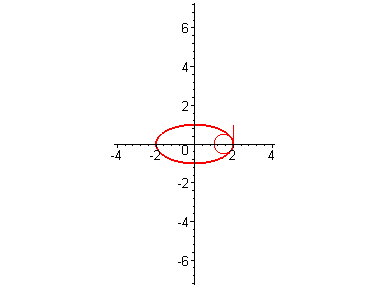Example 3 . Coefficient of engagement of a curve with the point.

IC of an ellipse is equal to 2; for the Pascal's limacon (it has one loop), IC= 4holds.

> restart: with(plots):

```Warning, existing definition for changecoords has been overwritten
```

> a:=1: b:=.2: r:=[(a*cos(t)-b)*cos(t), (a*cos(t)-b)*sin(t)]: t1:=0: t2:=2*Pi: # derive curvature of the limacon using the previous program

> rt:=diff(r, t): rt2:=diff(rt, t): rt3:=diff(rt2, t):

> k:=simplify((rt*rt2-rt*rt2)/sqrt(rt^2+rt^2)^3);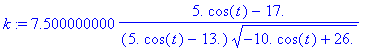> evalf(int(k, t=0..2*Pi)/(2*Pi));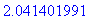> plot([k, diff(k,t)], t=0..2*Pi);>

Example 4 . The coefficient of engagement of a plane curve

In the program the curve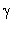_R under increasing R will intersect the origin n= 5 times.

> n:=5: f:=randpoly(z, terms=4, degree=n)+10000;> f1:=simplify(subs(z=R*(cos(t)+I*sin(t)), f)): f2:=collect(subs(I=y,f1), y): fy:=coeff(f2, y, 1); fx:=coeff(f2, y, 0);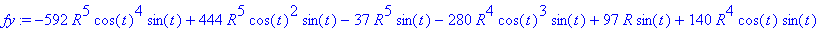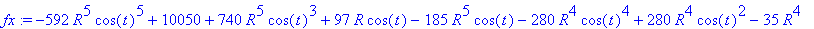> plots[animate]([fx, fy, t=0..2*Pi], R=0..3, numpoints=200,scaling=constrained);>

12.3 Curvature and Torsion of a Space Curve

The program for deriving of characteristics of the space curve at the given point.

> restart: with(linalg): Digits:=2; # Data.

```Warning, protected name norm has been changed and unprotected
```

```Warning, protected name trace has been changed and unprotected
```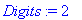> x:=t -> R*cos(t): y:=t -> R*sin(t): z:=t-> a*t: t0:=1: x0:=evalf(limit(x(t),t=t0));z0:=limit(z(t),t=t0); # Calculations by formulas at the point t.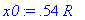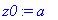> assume(R>0, a>0,t>0,t<Pi/2): r:=[x(t), y(t), z(t)]; rt:=diff(r, t); rt2:=diff(rt, t); rt3:=diff(rt2, t); tm:=[(x1-x(t))/rt,(y1-y(t))/rt,(z1-z(t))/rt]; p1:=simplify(det([[x1-x(t),y1-y(t),z1-z(t)], rt, rt2]))=0; tau:=evalm(rt/norm(rt, 2)); b1:=crossprod(rt, rt2); b:=evalm(b1/norm(b1,2)); n:=crossprod(b, tau); p2:=simplify(dotprod(tau, [x1-x(t),y1-y(t),z1-z(t)], 'orthogonal'))=0; p3:=simplify(dotprod(n, [x1-x(t), y1-y(t), z1-z(t)], 'orthogonal'))=0; k:=simplify(norm(b1,2)/norm(rt, 2)^3); kappa:=simplify(det([rt,rt2,rt3])/norm(b1, 2)^2);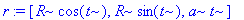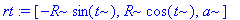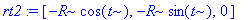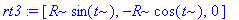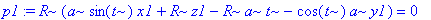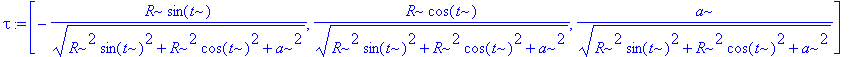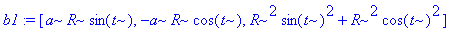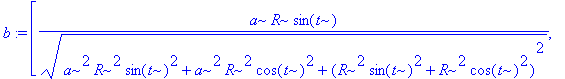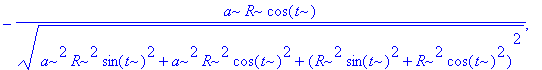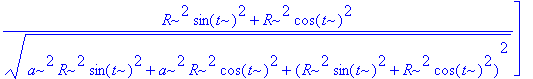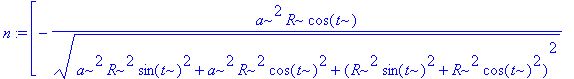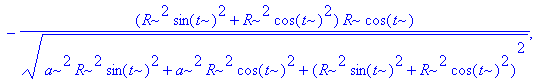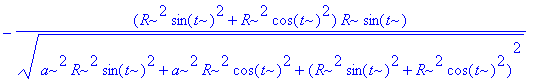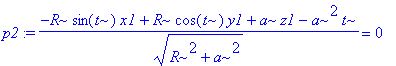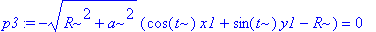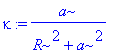Calculations at the given point t_0.

> t:=t0; tm0:=simplify(tm); p10:=simplify(p1);tau0:=evalm(tau); b0:=evalm(b); n0:=evalm(n);p20:=simplify(p2); p30:=simplify(p3); k0:=simplify(k); kappa0:=simplify(kappa);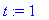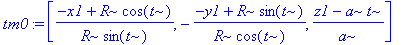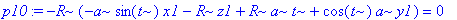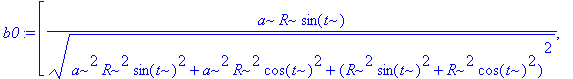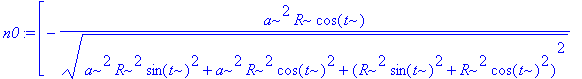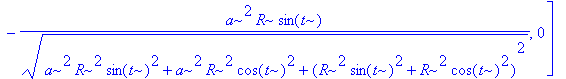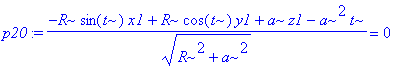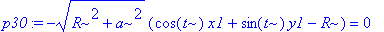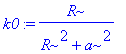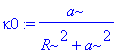>

Example 1 . Plotting the moving Frenet frame and osculating circles of a space curve,

with the test example of the curve of Viviani.

F.1 denotes the tangent vector; F.2 denotes the main normal vector; F.3 denotes the binormal vector;

F.5 denotes the osculating circle; F.4 denotes the segment of its position vector.

> restart: with(plots): with(linalg): R:=2: m:=32: t1:=0: t2:=2*Pi:

```Warning, existing definition for changecoords has been overwritten
```

```Warning, protected name norm has been changed and unprotected
```

```Warning, protected name trace has been changed and unprotected
```

> r:=[R*cos(t)^2, R*cos(t)*sin(t), R*sin(t)]:

> rt:=diff(r, t): rt2:=diff(rt, t): rt3:=diff(rt2, t): tau:=evalm(rt/norm(rt, 2)): b1:=crossprod(rt, rt2): b:=evalm(b1/norm(b1, 2)): n:=crossprod(b, tau):

> k:=simplify(norm(b1,2)/norm(rt,2)^3): F||1:=evalm(r+s*tau): F||2:=evalm(r+s*n): F||3:=evalm(r+s*b): F||4:=evalm(r+s*n/k): F||5:=evalm(r+n/k+(n*cos(2*Pi*s)+tau*sin(2*Pi*s))/k):

> T:=i->t1+i*(t2-t1)/m: for j from 1 to 5 do p||j:=i -> spacecurve(subs(t=T(i), evalm(F||j)), s=0..1): A||j:=display([seq(p||j(i), i=0..m)], insequence=true) od:

> B:=spacecurve(r, t=t1..t2, thickness=2):

> display([A||1, A||2, A||3, B], scaling=constrained, orientation=[40, 80], axes=framed);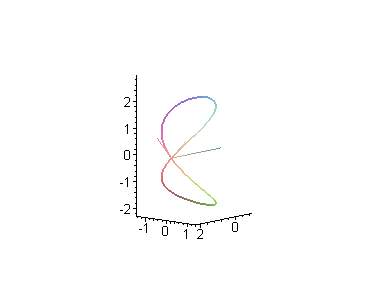> display([A||4, A||5, B], scaling=constrained, orientation=[40,80], axes=boxed);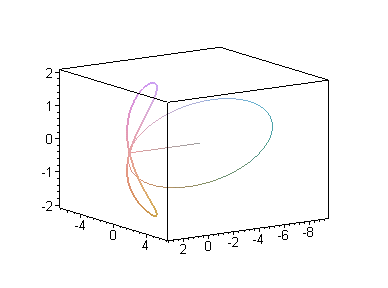>

12.4 Natural Equations of a Curve

By Theorem 12.4.1 the curve r(t) is not regular.

> f:=t^2*sin(2*Pi/t^2); ft:=diff(f, t);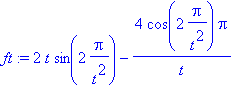> plot(f, t=0.2..2.5); plot(ft, t=0.45..2.5);>

Example 2 . The curve r(t) in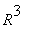of the class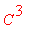that does not belong to the plane and whose curvature

k(t) vanishes at only one point, but with torsion(t)= 0.

> reaflib(piecewise):

> X:=t:Y:=piecewise(t<0,0,t^4):Z:=piecewise(t<0,t^4,0):

> plots[tubeplot]([X,Y,Z,radius=.1],t=-1.3..1.3, style=patchnogrid,scaling=constrained,axes=normal);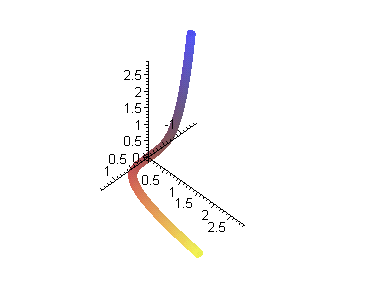>